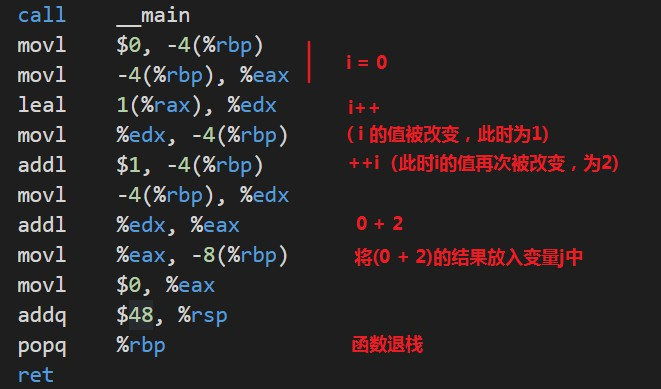# 小拓展：C语言中int的正确使用姿势

#include<stdio.h>
#include<stdint.h>

int main(void){
// 使用stdint.h中定义的类型表示整数
int8_t a = 0;
int16_t b = 0;
int32_t c = 0;
int64_t d = 0;

// 前面加u，表示unsigned,无符号
uint32_t e = 0;
printf("int8 size is %d\n",sizeof(int8_t));
printf("int16 size is %d\n",sizeof(int16_t));
printf("int32 size is %d\n",sizeof(int32_t));
printf("int64 size is %d\n",sizeof(int64_t));
printf("uint32 size is %d\n",sizeof(uint32_t));
}


int8 size is 1
int16 size is 2
int32 size is 4
int64 size is 8
uint32 size is 4


int8_t即表示8位整型，同理，int64_t就是64位整型，类型定义明确清晰，且能兼容多种平台。以上代码，使用32位编译器，编译成32位系统下的程序后，运行得到的结果依然不变。这里一定会有朋友质疑，为什么32位的系统下，还能表示并使用int64这种64位的整型？这当然就是stdint.h库给我们带来的便利了，简单说一下原理，如果当前平台的是32位的，那么经过组合，我们可以使用两个32位拼起来，不就能表示64位了吗？同理，即使是8位的CPU，经过这种拼合思路，照样能表示64位！当然，聪明人一眼就看出了弊端，使用这种拼合的方式，数据需要经过组合转换，处理也更加复杂，同时还会带来性能的损失，但是C99标准库已经为我们处理好了一切，虽然付出了一定的性能损失，但是成功的实现了C语言整型的跨平台兼容，这样的损失是完全值得的。

# 语法基础

## 表达式

### 算术运算符

+-*/

++--

int i = 0;
i++;
++i;


i++作为一个表达式，那么他的表达式的值是什么呢？其实我们可以用一个变量来保存表达式的值int r = i++;

    int i = 0;
int r = i++;

printf("r=%d\n",r);


    int i = 0;
int r = ++i;

printf("r=%d\n",r);


1. ++作为后缀时，自增表达式整体的值等于该变量初始值。如上例中int r = i++;，表达式整体的返回值r 等于i的初始值，而i未做自增运算前的初始值是0，所以r就是0。但是要注意，表达式一旦运行，i的值就会立刻发生变化，因此(i++)中，i的值是1

2. ++作为前缀时，自增表达式整体的返回值等于该变量运算之后的值。如上例中int r = ++i;r的值等于(++i)表达式运算之后i的实际值。

    int i = 0;
int j = i++ + ++i;
printf("i=%d, j=%d\n",i,j);


int main(void){
int i = 0;
i++ + ++i;
return 0;
}


gcc -S test.c


	call	__main
movl	$0, -4(%rbp) movl -4(%rbp), %eax addl$1, %eax
movl	%eax, -4(%rbp)
addl	$1, -4(%rbp) movl$0, %eax
addq	$48, %rsp popq %rbp ret  这里call __main相当于main函数入口，ret相当于return 0，这之间一段也就对应我们的两行C语言代码。特别说明一下，这里使用的gnu的工具链生成的是AT&T的x86-64汇编代码，而非大家熟悉的intel 80386汇编。高校教的汇编语言都是intel x86的32位汇编，因此学过汇编的人可能也会感觉非常陌生。实际上这段汇编非常简单，并不需要有什么汇编基础。 简单解释一下指令 movl 对应80386汇编中的mov指令，是单词move的缩写，表示传递数据，addl则对应add指令，表示加法器。这里的-4(%rbp)表示的是一个内存地址，eax则是32位对应的8个寄存器中的第一个。 movl$0, -4(%rbp)这句表示把一个常量0存到一个内存地址中，对应int i = 0;此后，-4(%rbp)这个地址就代指变量i
movl -4(%rbp), %eax这句表示将变量i中的值取出来放到一个名叫eax的寄存器中。addl $1, %eax则对应i++，表示将常量1与寄存器eax的值相加，然后存到eax中，那么此时eax的值就是1。紧接着movl %eax, -4(%rbp)，表示将寄存器eax的值刷新到变量i中，故而i++后，i的值立刻发生改变。 然后是addl$1, -4(%rbp)，这句对应的C语言代码是++i，它表示将常量1直接与变量i的值相加，结果仍然保存到变量i中，那么此时就是1+1，故而变量i最后等于2。

int main(void){
int i = 0;
int j = i++ + ++i;
return 0;
}int main(void){
int i = 0;
int j = 0;

i++;
++j;
}


	call	__main
movl	$0, -4(%rbp) movl$0, -8(%rbp)
addl	$1, -4(%rbp) addl$1, -8(%rbp)
movl	$0, %eax addq$48, %rsp
popq	%rbp
ret


### 关系运算符

<<=>>===

### 逻辑运算符

&&||!

### 赋值运算符

=表示赋值运算符，在C语言中，存在左值右值的概念。简单说，=左边的叫左值，右边的叫右值。左值只能是计算机内存中的对象，而不能是常量或计算的结果。例如变量可以成为左值，而像5i + 2这样的不能做左值。

int i,j,k;

i = j = k = 0;


=遵循右结合，所有它等价于i = (j = (k = 0))，也就是说0先赋值给k，然后k的值再赋值给j，以此类推。Ok，这样是没问题的。

    int i;
float j;
j = i = 6.1f;


j最终的值变成了6.0，这就是赋值陷阱。也就是说=存在类型自动转换的问题，值传递给i时，自动转化为int型，丢弃了小数部分。

    int8_t a = 0;
int16_t b = 0;
int32_t c = 0;
int64_t d = 0;

a += 1;  // 等价于 a = a + 1
b -=1;   // 等价于 a = a - 1
c *= 1;  // 等价于 a = a * 1
d /=2;   // 等价于 a = a / 1
d %=2;   // 等价于 a = a % 1


### 运算符优先级

1自增自减（后缀）i++i--
2自增自减（前缀）++i--i
3乘法类* / %
4加法类+ -
5赋值类= += -= ……

## 分支与循环

### 条件分支

C语言的条件分支与其他语言相似
if-else分支，如下结构，这是Linux C语言推荐的代码范式，即将一个花括号紧跟小括号之后，写在同一行。

if (1 > 0){
// do something
}else{
// do something
}


if后面的条件表达式中存在陷阱，在C语言中没有布尔类型，使用0和非0来表示false和true。因此很多人会想当然的以为0是false，大于0就是true，实际上，-1也是true，要注意，是一切非0值，包括小数也是true。

if-else中只有一句时，语法上是可以省略花括号的，但是不建议这样，尤其包含嵌套的if语句时。C语言语法比较自由，正是如此，才更应该遵守规范。始终写上花括号，养成良好的编程规范，使代码易于阅读和维护。

if(a>b) max=a;
else max=b;

// 或者放两行
if(a>b)
max=a;
else
max=b;


if(/*条件1*/){
//语句块1
} else  if(/*条件2*/){
//语句块2
} else  if(/*条件3*/){
//语句块3
}else{
//语句块n
}


int a = 1;

switch(a){
case 1:
printf("Monday\n");
break;
case 2:
printf("Tuesday\n");
break;
case 3:
printf("Wednesday\n");
break;
case 4:
printf("Thursday\n");
break;
case 5:
printf("Friday\n");
break;
case 6:
printf("Saturday\n");
break;
case 7:
printf("Sunday\n");
break;
default:
printf("error\n");
break;
}


### 循环

#### while

while(/*表达式*/){
//语句块
}

int i=1, sum=0;
while( i<=100 ){
sum+=i;
i++;
}


do{
//语句块
}while(/*表达式*/);

//---------------------------------
int i=1, sum=0;

do{
sum+=i;
i++;
}while(i<=100);


do-while循环与while循环的不同在于，它会先执行“语句块”，然后再判断表达式是否为真，如果为真则继续循环；如果为假，则终止循环。因此，do-while 循环至少要执行一次“语句块”。再使用do-while循环时，要记住，while(i<=100);的小括号后面必须跟一个分号。

#### for

C语言中更常用的可能是for循环

for 循环的一般形式

for(表达式1; 表达式2; 表达式3){
语句块
}

1. 先执行“表达式1”。
2. 再执行“表达式2”，如果它的值为真（非0），则执行循环体，否则结束循环。
3. 执行完循环体后再执行“表达式3”。
4. 重复执行步骤 2 和 3，直到“表达式2”的值为假，就结束循环。
// 使用for循环，进行等差数列求和
int sum=0;
for(int i=1; i<=100; i++){
sum+=i;
}

printf("%d\n",sum);


for 循环中的三个表达式都是可选项，都可以省略，但分号必须保留。

int i = 1, sum = 0;
for( ; i<=100; i++){
sum+=i;
}

// 省略两个
for( ; i<=100 ; ){
sum=sum+i;
i++;
}

// 全部省略，表示死循环，等同于while(1){}
for( ; ; ){
// do something
}


//表达式1 和 表达式3都是一个逗号表达式，即用逗号连接了两个表达式。
for( i=0,j=100; i<=100; i++,j-- )  k=i+j;


#### 控制循环

break 关键字用于 while、for 循环时，会终止循环而执行整个循环体后面的代码。break 关键字通常和 if 语句一起使用，即满足条件时便跳出循环

int i=1, sum=0;
while(1){  //死循环
sum+=i;
i++;
if(i>100) break;  //满足条件退出循环
}


continue 的作用是跳过本次循环中剩余的语句而强制进入下一次循环。它只用在 while、for 循环中，常与 if 条件语句一起使用

// 打印奇数
for(int i=1; i<=100; i++){
if(i%2 == 0){   // 遇到偶数时跳过
continue;
}
printf("%d\n",i);
}


# 欢迎关注我的公众号：编程之路从0到1“编程之路从0到1”
08-30461401-11643
05-241751
07-07404
02-231009
03-231万+
12-2382
06-241322
06-13895
12-142695
10-021245
11-251550
12-31
©️2020 CSDN 皮肤主题: 技术黑板 设计师:CSDN官方博客